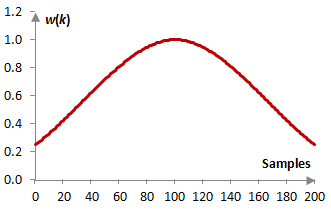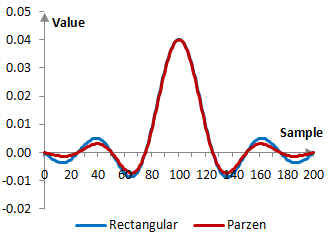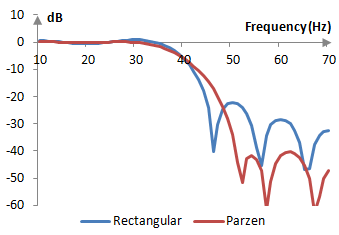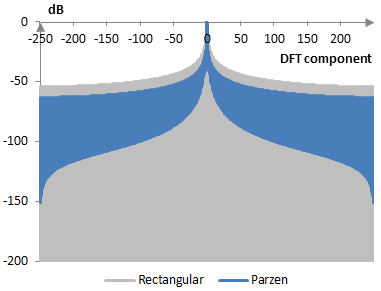# Parzen window

The Parzen window coefficients are given by the following formula

$$w(k)=\begin{cases} 1-6(\frac{|k-M|}{N})^2+6(\frac{|k-M|}{N})^3, |k-M| \le M \\ 2(1-\frac{|k-M|}{N})^3, |k-M| > M \end{cases}$$

where N is the length of the filter, M = (N – 1) / 2, and k = 0, 1, …, N – 1.

The Parzen window is a basic spline or B-spline window (see Triangular window). It is the fourth order convolution of the rectangular window with itself.

Take a finite impulse response (FIR) low pass filter of length N = 201. The following is the Parzen window.Given a sampling frequency of 2000 Hz and a filter cutoff frequency of 40 Hz, the impulse response of the filter with a rectangular window (with no window) and with the Parzen window is as follows.The magnitude response of the same filter is shown on the graph below.## Measures for the Parzen window

The following graph compares the discrete Fourier transform of the Parzen window with that of the rectangular window.The Parzen window measures are as follows.

 Coherent gain 0.69 Equivalent noise bandwidth 1.12 Processing gain -0.50 dB Scalloping loss -2.57 dB Worst case processing loss -3.07 dB Highest sidelobe level -24.0 dB Sidelobe falloff -7.0 dB / octave, -23.3 dB / decade Main lobe is -3 dB 1.08 bins Main lobe is -6 dB 1.48 bins Overlap correlation at 50% overlap 0.393 Amplitude flatness at 50% overlap 0.869 Overlap correlation at 75% overlap 0.792 Amplitude flatness at 75% overlap 0.966

Window

### Filtered HTML

• Freelinking helps you easily create HTML links. Links take the form of [[indicator:target|Title]]. By default (no indicator): Click to view a local node.